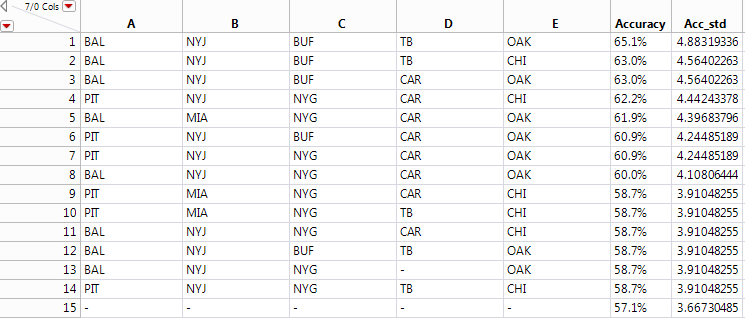Choose Language Hide Translation Bar

## How do I have tabulate iterate through multiple columns using JSL

I'm pretty new to JSL and I'm stuck on something that seems like it should work, but it isn't.  This is what I'm trying to create:Here's what the dataset looks like (well, it's fake, but you get the point):and this is the code I'm trying to use:

top15<<Tabulate(

Column Table( Analysis Columns( :Acc_std ) ),

for(i=1,

i<=nitems(top15<<get column names())-1,

i++,

Row Table( Grouping Columns( column name(i) ) )

)

)

);

I'm doing it this way because when I created the tabulate manually the script output was:

Tabulate(

Column Table( Analysis Columns( :Acc_std ) ),

Row Table( Grouping Columns( :A ) ),

Row Table( Grouping Columns( :B ) ),

Row Table( Grouping Columns( :C ) ),

Row Table( Grouping Columns( ) ),

Row Table( Grouping Columns( :E ) )

)

);

instead of writing out each column, I was hoping to just be able to iterate through the columns (using the for loop). Where am I going wrong, or am I completely going down the wrong path here?

1 ACCEPTED SOLUTION

Accepted Solutions

## Re: How do I have tabulate iterate through multiple columns using JSL

You can either build the required expression in advance (see, for example https://community.jmp.com/message/224888#224888), or add the row tables incrementally, as below.

NamesDefaultToHere(1);

// Example table

dt = New Table( "Test",

New Column( "A",

Numeric,

"Nominal",

Format( "Best", 12 ),

Formula( Random Integer( 4 ) )

),

New Column( "B",

Numeric,

"Nominal",

Format( "Best", 12 ),

Formula( Random Integer( 5 ) )

),

New Column( "Y",

Numeric,

"Continuous",

Format( "Best", 12 ),

Formula( Random Normal() )

)

);

// List of grouping columns

cList = {:A, :B};

// Tabulate

tab = dt << Tabulate(Add Table( Column Table( Analysis Columns( :Y ) )));

// Add grouping columns in cList

For (c=1, c<=NItems(cList), c++,

tab << AddTable(Row Table( Grouping Columns( cList[c] ) ));

);

I also note that (for some reason) when I cut and paste the above code into the 'Reply' box the 'Analysis Column' was switched from ':Y' to ':And' (!?)

4 REPLIES 4

## Re: How do I have tabulate iterate through multiple columns using JSL

You can either build the required expression in advance (see, for example https://community.jmp.com/message/224888#224888), or add the row tables incrementally, as below.

NamesDefaultToHere(1);

// Example table

dt = New Table( "Test",

New Column( "A",

Numeric,

"Nominal",

Format( "Best", 12 ),

Formula( Random Integer( 4 ) )

),

New Column( "B",

Numeric,

"Nominal",

Format( "Best", 12 ),

Formula( Random Integer( 5 ) )

),

New Column( "Y",

Numeric,

"Continuous",

Format( "Best", 12 ),

Formula( Random Normal() )

)

);

// List of grouping columns

cList = {:A, :B};

// Tabulate

tab = dt << Tabulate(Add Table( Column Table( Analysis Columns( :Y ) )));

// Add grouping columns in cList

For (c=1, c<=NItems(cList), c++,

tab << AddTable(Row Table( Grouping Columns( cList[c] ) ));

);

I also note that (for some reason) when I cut and paste the above code into the 'Reply' box the 'Analysis Column' was switched from ':Y' to ':And' (!?)

## Re: How do I have tabulate iterate through multiple columns using JSL

That worked perfectly!

Just so that I understand, it looks like you named the tabulate window "tab" and that allowed you to send additional commands to it, correct?

## Re: How do I have tabulate iterate through multiple columns using JSL

Yes. JMP reports and their constituent parts are essentially objects that you can send messages to. By assigning a reference to an object (in this case 'tab'), you can then do 'Show Properties(tab)' to see what messages that object knows about and can respond to.

Take a look at chapters 10 and 11 of the Scripting Guide ('Help > Books > Scripting Guide') and also 'Help > Scripting Index'.